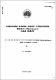## Calculation methods for multigroup neutron cross sections used in burnup studies1967-10
##### Publisher
Australian Atomic Energy Commission
##### Abstract
Calculation of burnup of material composing a nuclear reactor is important in a feasibility study of lifetime of fuel in possible reactor systems. This work discusses (i) a basis for calculating a flux spectrum in order to obtain multigroup cross sections and (ii) a method for solving the burnup equations. Under (i) particular attention is paid to the calculation of multigroup resonance cross sections and (ii) a simple and effective analytic technique is derived- In addition, this work presents, in a consistent manner, developments in the field of reactor physics which effect the calculation of multigroup data. The physical model adopted to simplify the otherwise unmanageable equations is appropriate to a large recirculating fuel reactor operating under equilibrium conditions. The work is divided into essentially three parts (i) the basis of multigroup data (Sections 2 and 3), (ii) the calculation of multigroup data (Sections 4, 5 and 6) and (iii) the solution of burnup and multigroup flux equations (Sections 7 and 8). Considering that neutron reactions are very dependent on neutron energy it is convenient to divide the energy range into three regions typified by completely different behaviour. These are (a) the fast region (Section 4) — neutrons are produced from fission, (b) the resonance region (Section 5) — neutrons slow down in energy and (c) the thermal region (Section 6) — neutrons scatter up and down in energy until lost
##### Keywords
Cross sections, Neutrons, Burnup, Diffusion
##### Citation
Pollard, J. P. (1967). Calculation methods for multigroup neutron cross sections used in burnup studies (AAEC/E184). Lucas Heights, NSW: Australian Atomic Energy Commission.
Neutron diffusion equation